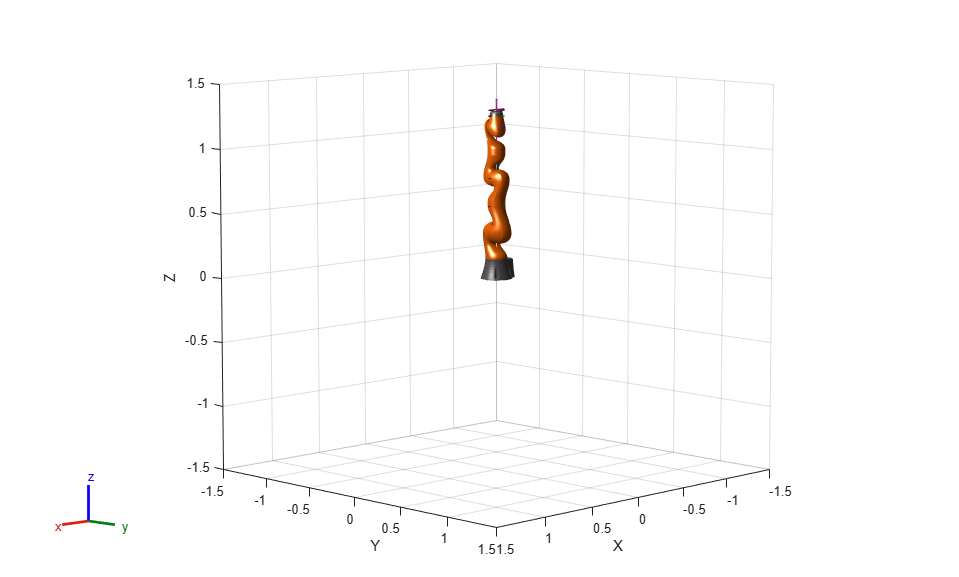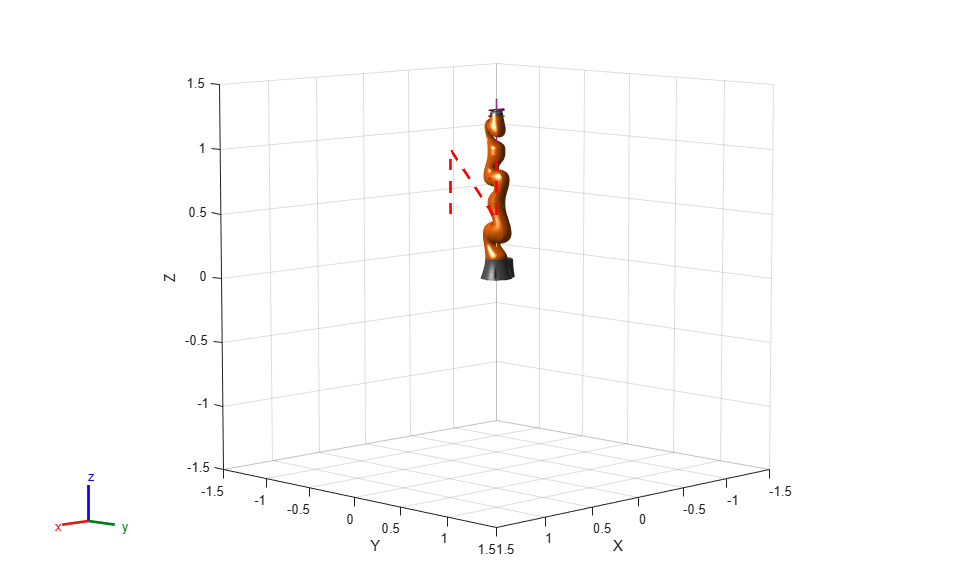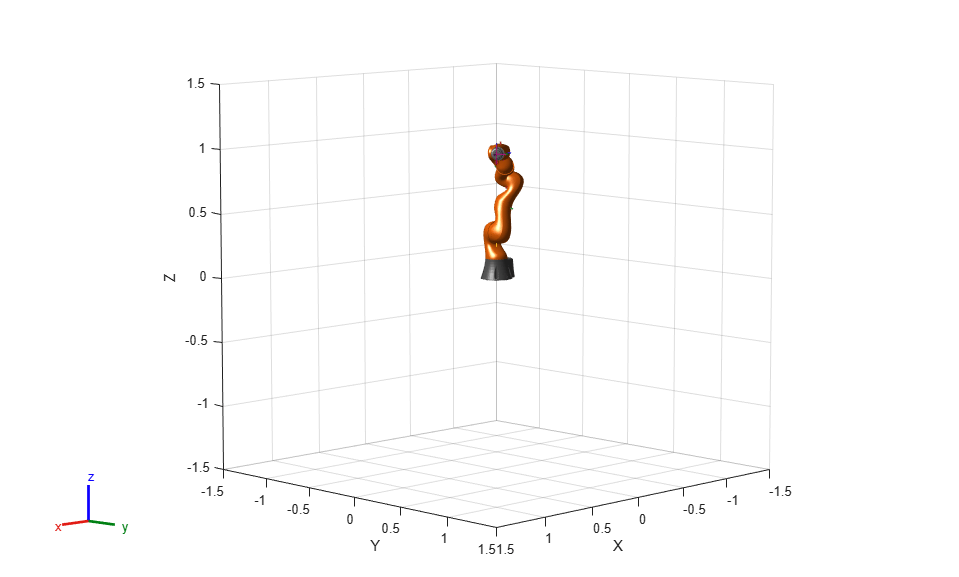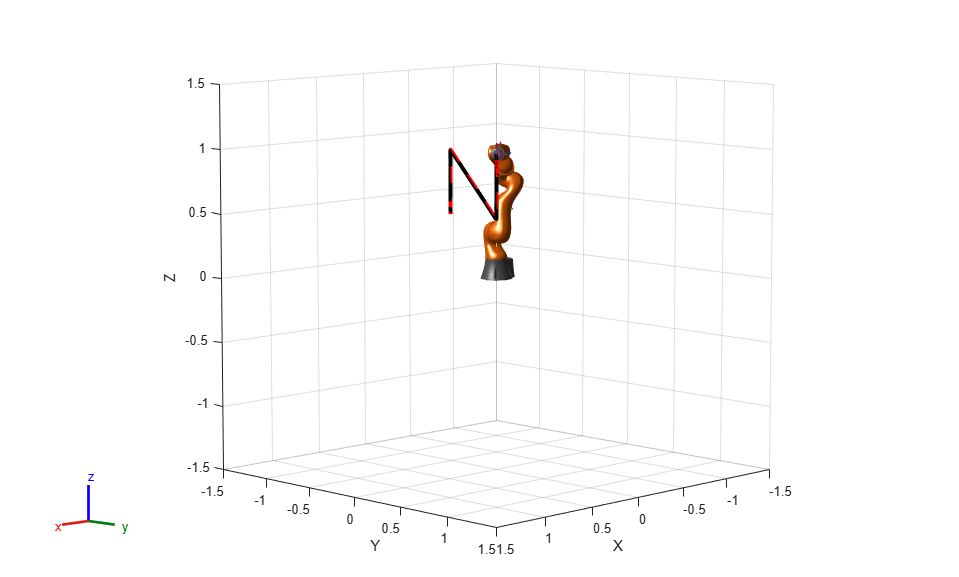# Trace An End-Effector Trajectory with Inverse Kinematics in Simulink

Use a rigid body robot model to compute inverse kinematics using Simulink®. Define a trajectory for the robot end effector and loop through the points to solve robot configurations that trace this trajectory.

Import a robot model from a URDF (unified robot description format) file as a `RigidBodyTree` object.

```robot = importrobot('iiwa14.urdf'); robot.DataFormat = 'column'; ```

View the robot.

```ax = show(robot); ```Specify a robot trajectory. These xyz-coordinates draw an N-shape in front of the robot.

```x = 0.5*zeros(1,4)+0.25; y = 0.25*[-1 -1 1 1]; z = 0.25*[-1 1 -1 1] + 0.75; hold on plot3(x,y,z,'--r','LineWidth',2,'Parent',ax) hold off ```Open a model that performs inverse kinematics. The xyz-coordinates defined in MATLAB® are converted to homogeneous transformations and input as the desired `Pose`. The output inverse-kinematic solution is fed back as the initial guess for the next solution. This initial guess helps track the end-effector pose and generate smooth configurations.

You can press the callback button to regenerate the robot model and trajectory you just defined.

```close open_system('sm_ik_trajectory_model.slx') ```Run the simulation. The model should generate the robot configurations (`configs`) that follow the specified trajectory for the end effector.

```sim('sm_ik_trajectory_model.slx') ```

Loop through the robot configurations and display the robot for each time step. Store the end-effector positions in `xyz`.

```figure('Visible','on'); tformIndex = 1; for i = 1:10:numel(configs.Data)/7 currConfig = configs.Data(:,1,i); show(robot,currConfig); drawnow xyz(tformIndex,:) = tform2trvec(getTransform(robot,currConfig,'iiwa_link_ee')); tformIndex = tformIndex + 1; end ```Draw the final trajectory of the end effector as a black line. The figure shows the end effector tracing the N-shape originally defined (red dotted line).

```figure('Visible','on') show(robot,configs.Data(:,1,end)); hold on plot3(xyz(:,1),xyz(:,2),xyz(:,3),'-k','LineWidth',3); plot3(x,y,z,'--r','LineWidth',3) hold off ```